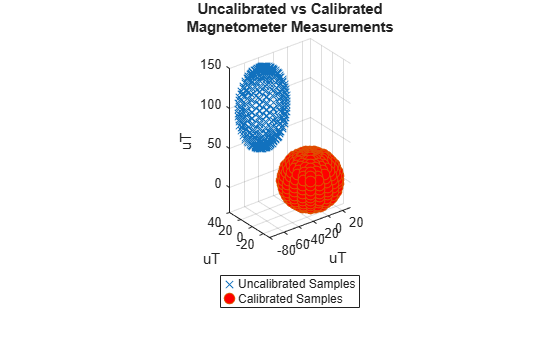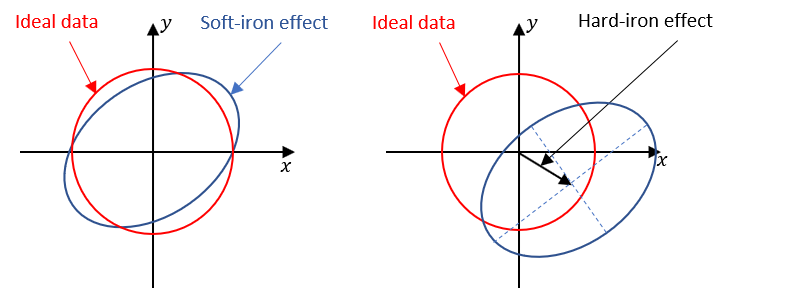Main Content

# magcal

Magnetometer calibration coefficients

## Syntax

``[A,b,expmfs] = magcal(D)``
``[A,b,expmfs] = magcal(D,fitkind)``

## Description

example

````[A,b,expmfs] = magcal(D)` returns the coefficients needed to correct uncalibrated magnetometer data `D`.To produce the calibrated magnetometer data `C`, use equation `C = (D-b)*A`. The calibrated data `C` lies on a sphere of radius `expmfs`.```
````[A,b,expmfs] = magcal(D,fitkind)` constrains the matrix `A` to be the type specified by `fitkind`. Use this syntax when only the soft- or hard-iron effect needs to be corrected.```

## Examples

collapse all

Generate uncalibrated magnetometer data lying on an ellipsoid.

```c = [-50; 20; 100]; % ellipsoid center r = [30; 20; 50]; % semiaxis radii [x,y,z] = ellipsoid(c(1),c(2),c(3),r(1),r(2),r(3),20); D = [x(:),y(:),z(:)];```

Correct the magnetometer data so that it lies on a sphere. The option for the calibration is set by default to 'auto'.

```[A,b,expmfs] = magcal(D); % calibration coefficients expmfs % Dipaly expected magnetic field strength in uT```
```expmfs = 31.0723 ```
`C = (D-b)*A; % calibrated data`

Visualize the uncalibrated and calibrated magnetometer data.

```figure(1) plot3(x(:),y(:),z(:),'LineStyle','none','Marker','X','MarkerSize',8) hold on grid(gca,'on') plot3(C(:,1),C(:,2),C(:,3),'LineStyle','none','Marker', ... 'o','MarkerSize',8,'MarkerFaceColor','r') axis equal xlabel('uT') ylabel('uT') zlabel('uT') legend('Uncalibrated Samples', 'Calibrated Samples','Location', 'southoutside') title("Uncalibrated vs Calibrated" + newline + "Magnetometer Measurements") hold off```## Input Arguments

collapse all

Input matrix of raw magnetometer data, specified as a N-by-3 matrix. Each column of the matrix corresponds to the magnetometer measurements in the first, second and third axes, respectively. Each row of the matrix corresponds to a single three-axis measurement.

Data Types: `single` | `double`

Matrix type for output `A`. The matrix type of `A` can be constrained to:

• `'eye'` – identity matrix

• `'diag'` – diagonal

• `'sym'` – symmetric

• `'auto'` – whichever of the previous options gives the best fit

## Output Arguments

collapse all

Correction matrix for the soft-iron effect, returned as a 3-by-3 matrix.

Correction vector for the hard-iron effect, returned as a 3-by-1 array.

Expected magnetic filed strength, returned as a scalar.

## More About

collapse all

### Soft- and Hard-Iron Effects

Because a magnetometer usually rotates through a full range of 3-D rotation, the ideal measurements from a magnetometer should form a perfect sphere centered at the origin if the magnetic field is unperturbed. However, due to distorting magnetic fields from the sensor circuit board and the surrounding environment, the spherical magnetic measurements can be perturbed. In general, two effects exist.

1. The soft-iron effect is described as the distortion of the ellipsoid from a sphere and the tilt of the ellipsoid, as shown in the left figure. This effect is caused by disturbances that influence the magnetic field but may not generate their own magnetic field. For example, metals such as nickel and iron can cause this kind of distortion.

2. The hard-iron effect is described as the offset of the ellipsoid center from the origin. This effect is produced by materials that exhibit a constant, additive field to the earth's magnetic field. This constant additive offset is in addition to the soft-iron effect as shown in the figure on the right.The underlying algorithm in `magcal` determines the best-fit ellipsoid to the raw sensor readings and attempts to "invert" the ellipsoid to produce a sphere. The goal is to generate a correction matrix `A` to account for the soft-iron effect and a vector `b` to account for the hard-iron effect. The three output options, 'eye', 'diag' and 'sym' correspond to three parameter-solving algorithms, and the 'auto' option chooses among these three options to give the best fit.

 Ozyagcilar, T. "Calibrating an eCompass in the Presence of Hard and Soft-iron Interference." Freescale Semiconductor Ltd. 1992, pp. 1-17.